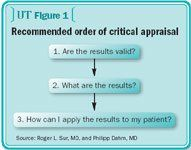# Interpreting journal articles: How to validate, apply results

It is incredibly tempting for readers to immediately look at the results of a study and determine the conclusions; however, the evidence-based mindset requires a more rational approach that questions the validity of the results, followed by a close inspection of them.

We previously discussed how to determine the validity of a therapy article and whether to trust the results (see, "Interpreting journal articles: Practical tips for urologists," Urology Times, Nov. 2007, pg. 18-9). As that article explained, critical appraisal of a published study requires that we first determine whether the results can be trusted, and only then should we look at the results (figure 1).What are the results?

How large was the treatment effect? Consider a two-arm treatment study involving a dichotomous outcome or event (ie, "yes," the event occurred, or "no," it did not occur), such as whether a drug decreases mortality, in a randomized controlled trial. Perhaps the group of patients who received the drug (x) had 6% mortality, while the placebo group (y) experienced 10% mortality. There are several ways to express these results. We could simply state that the drug decreased the risk of mortality by 4%; that is, the absolute risk reduction (ARR) = y–x (0.10–0.06 = 0.04). Another common method of expression is the relative risk (RR), whereby the ratio of risks is computed. Either group can be the numerator or denominator, such as x/y (0.06/0.10 = 0.60), which would be interpreted as a 60% risk of death using drug therapy compared to using placebo. This risk can be further expressed by a relative risk reduction (RRR), whereby the risk would be calculated as 1–RR or 1–x/y (1–0.60 = 0.40). The RRR would be expressed as a 40% decrease in mortality when using the drug therapy.